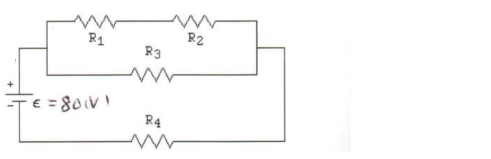# Problem: In the figure, consider the circuit sketched. The battery has emf ε = 80 volts and negligible internal resistance. The four resistors have resistances of R1 = 14 Ω, R2 = 21 Ω, R3 = 21 Ω, and R4 = 14 Ω. Find the voltage drop across R4.

🤓 Based on our data, we think this question is relevant for Professor Kang's class at UCF.

###### Problem Details

In the figure, consider the circuit sketched. The battery has emf ε = 80 volts and negligible internal resistance. The four resistors have resistances of R1 = 14 Ω, R2 = 21 Ω, R3 = 21 Ω, and R4 = 14 Ω. Find the voltage drop across R4